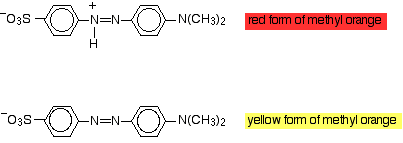# Why does methanol (an alcohol) make methyl orange (an indicator) turn yellow?

##### 1 Answer
Apr 20, 2016

See the explanation below.

#### Explanation:

Methyl orange is an intensely-coloured indicator that is red below $\text{pH 3.1}$ and orange-yellow above $\text{pH 4.4}$.The red (acid) form has an ${\text{H}}^{+}$ attached to one of the $\text{N}$ atoms.

The yellow (basic) form has lost the ${\text{H}}^{+}$.

The $\text{pH}$ of pure water ($\text{p"K_"a} = 15.7$) is 7.00. That is in the yellow region of the indicator range.

Methanol ($\text{p"K_"a} = 15.2$) is an even weaker acid than water.

Methanol is even less able than water to protonate the $\text{N}$ atom of methyl orange, so the indicator is yellow in methanol.